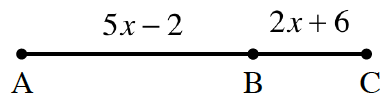### Home > INT1 > Chapter 3 > Lesson 3.1.1 > Problem3-10

3-10.

AC is a straight segment as shown in the diagram below. If the distance between points A and C is $67$ miles, determine the distance between points A and B.$5x − 2 + 2x + 6 = 67$